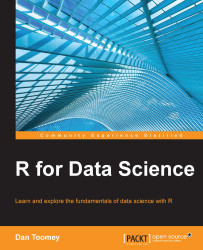•#### R for Data Science#### Overview of this book

R for Data ScienceCreditswww.PacktPub.comPrefaceFree Chapter
Data Mining PatternsData Mining SequencesText MiningData Analysis – Regression AnalysisData Analysis – CorrelationData Analysis – ClusteringData Visualization – R GraphicsData Visualization – PlottingData Visualization – 3DMachine Learning in ActionPredicting Events with Machine LearningSupervised and Unsupervised LearningIndex## Packages

We will use the following packages available in R for supervised and unsupervised learning:

• `rattle`: This is a data mining GUI in R

• `rpart.plot`: This is used to plot the `r.part` models

• `caret`: This is used for classification and regression training

• `kknn`: These are the weighted k nearest neighbors

• `kernlab`: This is used for kernel-based machine learning

• `e1071`: This package contains miscellaneous functions of the Department of Statistics

• `MCMCpack`: This package contains the functions for Markov chain Monte Carlo algorithm

• `randomForest`: This is used for classification and regression based on a forest of trees using random inputs

• `FactoMineR`: This is used for multivariate exploratory data analysis and data mining with R

### Supervised learning

In supervised learning we have a target variable and a number of possible predictor variables. The objective is to associate the predictor variables in such a way so as to accurately predict the target variable. We are using some portion of observed data to...